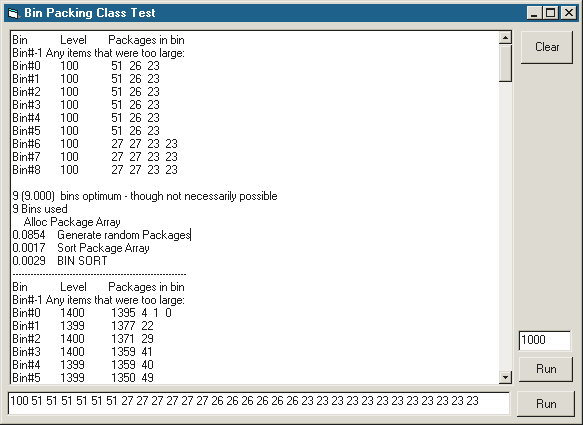# 1 Dimensional Bin Sorting Class

This class can perform a one dimensional bin packing. It could be used to: Sort packages of a certain weight into containers that can carry a given weight, cut 2x4s of a certain length into the required pieces with minimal waste, fill disks with a list of files… An example of a two dimensional bin packing is fitting images onto a page and a 3D example is fitting packages into a truck. A mix between standard Best Fit and Worst Fit algorithms is used. Data must be entered and sorted descending before calling the main PackBins Sub.Time Complexity (TC) of this function where

```N = # of items
B = min # of bins needed
= 1 + int(sum(1 to N) of itemsize / BinCapacity)
TC = N * B (i.e. we loop through the N items comparing most of them to each bin)```
```On my computer:
1000items-> ~200bins ==> 200,000 .06sec
5000items->~1000bins ==> 5,000,000 1.50sec```

If the average size of an item is 1/2 bin size then TC = N * N/2 or more generally:

```TC = N * N * Save%
TC = N 2 * Save%
(where S is the percentage of average item size relative to bin capacity)```

So if average item size doesn’t change doubling the number of items quadruples time spent. Using the second test time above would mean 5 million items would take a whopping 17.4 days to sort (5mil/5000 → 10002 * 1.5sec). However, we could assume that not much is gained by considering all items together. You could instead break it down to 5000 reps of the 1000 item problem which would give 0.06s * 5000 = 5min. You might save the results of each repetition, but remove the items in the last bin if not near full and return them to the items remaining to be sorted.

Also note that as S is decreased the number of bins needed will approach the optimum. And as S is increased the assumption above is less true; it becomes more important to check as many items as possible to reduce the number of bins needed. I lack the math skills to prove it, but it’s not difficult to see that if you have 1000 items with values ranging from 0 to 1000 and the bin capacity is 1000, there will be many bins with a large first item that won’t have a near perfect match which will result in extra bins needed. So given a maximum item size Smax there should be a point at which checking more items causes a huge time penalty without doing much to optimize the number of bins needed: Iopt = Smax * M (where if Save% is large M > 1).

One last note: It is perfectly possible that the number of bins needed far exceeds the predicted optimum without implying that the sort did a bad job. Consider a problem where bin capacity is 10 and you have 10 items of size 6. Total size divided by bin capacity (60/10) predicts that at least 6 bins will be needed while it is clear that 10 bins are needed.